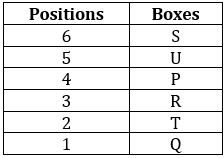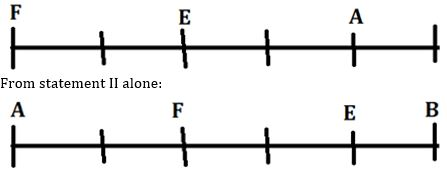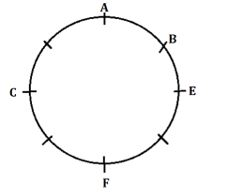Latest Banking jobs   »

# Reasoning Ability Quiz For IBPS RRB PO Clerk Mains 2022- 7th September

Directions (1-5): A word arrangement machine when given an input of words, rearranges the words following a particular set of rules. An illustration of that is given below.

Input: RAM TYU GTR WTI VFD DGH
Step I: DGH GTR RAM TYU VFD WTI
Step II: DGH GRT AMR TUY DFV ITW
Step III: EHI HSU ZNS UTZ EGW HUX
Step IV: ZNS UTZ HUX HSU EHI EGW
Step V: NSZ TUZ HUX HSU EHI EGW

Step V is the last step of the rearrangement. Following the same set of rules rearrange the input given below and answer the questions based on it.

Input: PQU GRB UTV XQJ WYK SHD

Q1. Which of the following is the last step of the given input?
(a) TUW QRT LXZ KRY EIT CHS
(b) TUW LXZ QRT KRY EIT CHS
(c) TUW QRT LXZ EIT KRY CHS
(d) TUW LXZ QRT KRY CHS EIT
(e) None of these

Q2. Which word is the third from the right end in step III of the given input?
(a) EIT
(b) UTW
(c) QRT
(d) LXZ
(e) None of these

Q3. In which step ‘QRT EIT UTW’ will be found in the same sequence?
(a) Step II
(b) Step IV
(c) Step III
(d) Step V
(e) None of these

Q4. What is the position of ‘QRT’ from right end in the step IV of the given input?
(a) First
(b) Fourth
(c) Fifth
(d) Second
(e) None of these

Q5. Which of the following is the second last step of the given input?
(a) UTW QRT LXZ KRY EIT CHS
(b) TUW QRT LXZ KRY EIT CHS
(c) TUW LXZ QRT KRY CHS EIT
(d) UTW QRT LXZ EIT KRY CHS
(e) None of these

Directions (6-10): A number arrangement machine when given an input line of numbers rearranges them following a particular rule in each step. The following is an illustration of input and rearrangement.

Input: 14 13 19 7 26 22 21
Step I: 21 20 26 14 33 29 28
Step II: 121 100 256 16 529 361 324
Step III: 12 3 39 21 48 30 27
Step IV: 150 15 1527 447 2310 906 735
Step V: 12 12 30 30 12 30 30
Step VI: 11 11 11 29 29 29 29

Step VI is the last step of the above input. As per rules followed in the above steps, find out in each of the given questions the appropriate steps for the given input.

Given input: 17 11 15 16 18 9 13

Q6. What is the sum of the number which is third from the left end in third step and number which is second from the right end in the step VI?
(a) 57
(b) 56
(c) 47
(d) 59
(e) None of these

Q7. In which step ’27 48 27’ will be found in the same sequence?
(a) Step II
(b) Step IV
(c) Step III
(d) Step VI
(e) None of these

Q8. In step IV, which number is 4th to the right of the number which is 2nd from the left end?
(a) 2310
(b) 728
(c) 456
(d) 735
(e) 15

Q9. Which of the following number is 2nd from the left end in step II?
(a) 144
(b) 64
(c) 196
(d) 36
(e) None of these

Q10. What is the sum of the number which is 2nd from the left end and 3rd from the right end in step V?
(a) 60
(b) 52
(c) 42
(d) 50
(e) None of these

Directions (11-15): The question consists of two statements numbered I and II given below it. You have to decide whether the data provided in the statement is sufficient to answer the question.

Q11. Six boxes, P, Q, R, S, T and U are placed one above another, where the bottommost box is 1, the box above it is 2 and so on up to 6 which is placed at the topmost position. Which box ix placed just above R?
Statements: I. P is placed 2nd above of T which is placed just above of Q. Two boxes are placed between T and U.
II. S is placed above P. R is not placed at the even numbered position.
(a) Data given in both statements I and II together are sufficient to answer.
(b) Data given in statement I alone is sufficient to answer.
(c) Data given in statement II alone is sufficient to answer.
(d) Data given in both statements I and II together are not sufficient to answer.
(e) Data given in either statement I or statement II alone is sufficient to answer the question.

Q12. There are six persons namely, A to F of different ages. Who is the oldest person?
Statements: I. E is older than A who is older than C. F is younger than D.
II. D is older than E. B is not the oldest person.
(a) Data given in both statements I and II together are sufficient to answer.
(b) Data given in statement I alone is sufficient to answer.
(c) Data given in statement II alone is sufficient to answer.
(d) Data given in both statements I and II together are not sufficient to answer.
(e) Data given in either statement I or statement II alone is sufficient to answer the question.

Q13. Who sits second to the right of F if A, B, C, D, E and F are sitting in a straight row facing north but not necessarily in the same order?
Statements: I. Three persons sit between A and F who sits at the extreme left end of the row. A sits second to the right of E.
II. B sits third to the right of F who sits second to the right of A. Three persons sit between A and E.
(a) Data given in both statements I and II together are sufficient to answer.
(b) Data given in statement I alone is sufficient to answer.
(c) Data given in statement II alone is sufficient to answer.
(d) Data given in both statements I and II together are not sufficient to answer.
(e) Data given in either statement I alone or statement II alone is sufficient to answer the question.

Q14. Eight persons A, B, C, D, E, F, G, and H are sitting along a circular table facing towards the center of the table but not necessarily in the same order. Who sits second to the right of A?
Statements: I. Three persons sit between A and F who sits second to the left of E.
II. B sits third to the right of F who sits second to the right of C.
(a) Data given in both statements I and II together are sufficient to answer.
(b) Data given in statement I alone is sufficient to answer.
(c) Data given in statement II alone is sufficient to answer.
(d) Data given in both statements I and II together are not sufficient to answer.
(e) Data given in either statement I alone or statement II alone is sufficient to answer the question.

Q15. Eight persons P, Q, R, S, T, U, V, and W are sitting around a circular table facing the center. Who sits to the immediate right of W?
Statements: I. P sits third to the right of U. W sits second to the right of P.
II. R does not sit adjacent to S.
(a) Data given in both statements I and II together are sufficient to answer.
(b) Data given in statement I alone is sufficient to answer.
(c) Data given in statement II alone is sufficient to answer.
(d) Data given in both statements I and II together are not sufficient to answer.
(e) Data given in either statement I alone or statement II alone is sufficient to answer the question.

Solutions

Solution (1-5):
Step I: The words are arranged in the alphabetical order from the left end
Step II: The letters of the words are arranged in alphabetical order within the word.
Step III: The consonants are replaced with the next letter and the vowels are replaced with the previous letter of the English alphabetical series (within each word).
Step IV. The words are arranged in reverse alphabetical order from left end.
Step V: The letters of the word are arranged in alphabetical order within the word.

Input: PQU GRB UTV XQJ WYK SHD
Step I: GRB PQU SHD UTV WYK XQJ
Step II: BGR PQU DHS TUV KWY JQX
Step III: CHS QRT EIT UTW LXZ KRY
Step IV: UTW QRT LXZ KRY EIT CHS
Step V: TUW QRT LXZ KRY EIT CHS

S1. Ans.(a)
S2. Ans.(b)
S3. Ans.(c)
S4. Ans.(c)
S5. Ans.(a)

Solution (6-10):
Step I: Add 7 to each number of the input
Step II: Subtract 10 from each number and then square the obtained number.
Step III: Add all digits within the numbers and then multiply the obtained number by 3.
Step IV: Do the Square of each number of step III and then add 6.
Step V: Add all the digits within the numbers and multiply the obtained number by 2.
Step VI: Subtract each number by 1 and then arrange in ascending order.

Given input: 17 11 15 16 18 9 13
Step I: 24 18 22 23 25 16 20
Step II: 196 64 144 169 225 36 100
Step III: 48 30 27 48 27 27 3
Step IV: 2310 906 735 2310 735 735 15
Step V: 12 30 30 12 30 30 12
Step VI: 11 11 11 29 29 29 29

S6. Ans.(b)
S7. Ans.(c)
S8. Ans.(d)
S9. Ans.(b)
S10. Ans.(a)

S11. Ans. (a)
Sol. From statements I and II together:Hence, data given in both statements I and II together are sufficient to answer that P is placed just above R.

S12. Ans. (a)
Sol. From statements I and II together: D > E > A > C and D > F
Hence, data given in both statements I and II together are sufficient to answer that D is the oldest person.

S13. Ans. (e)
Sol. From statement I alone:Hence, data given in either statement I alone or statement II alone is sufficient to answer the question.

S14. Ans. (a)
Sol. From statements I and II together:Hence, data given in both statements I and II together are sufficient to answer.

S15. Ans. (d)
Sol. From both statements, we can’t get the answer. So, data given in both statements I and II together are not sufficient to answer.#### Congratulations!Download Hindu Review of October 2021: Free PDF